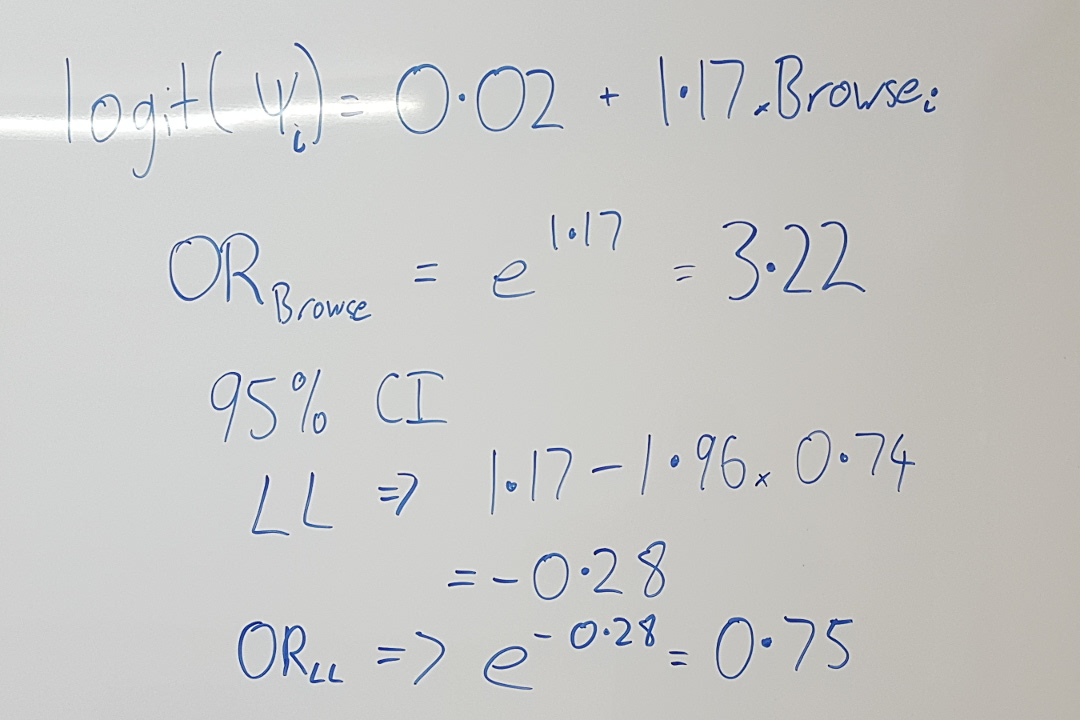When analysing data with logistic regression, or using the logit link-function to model probabilities, the effect of covariates and predictor variables are on the logistic-scale. These can easily be used to calculate odd ratios, which are commonly used to interpret effects using such techniques, particularly in medical statistics. In this video Darryl explains how you can calculate the odds ratio, as well calculations for associated confidence intervals estimates and standard errors.

For more on odds ratios, check out Darryl's 'beer fridge statistics' video. For more on confidence intervals watch this video about how to interpret them, and this one if comparing two intervals.

This video was requested by a viewer. Let us know if there's a statistical concept you'd like to see a video on!